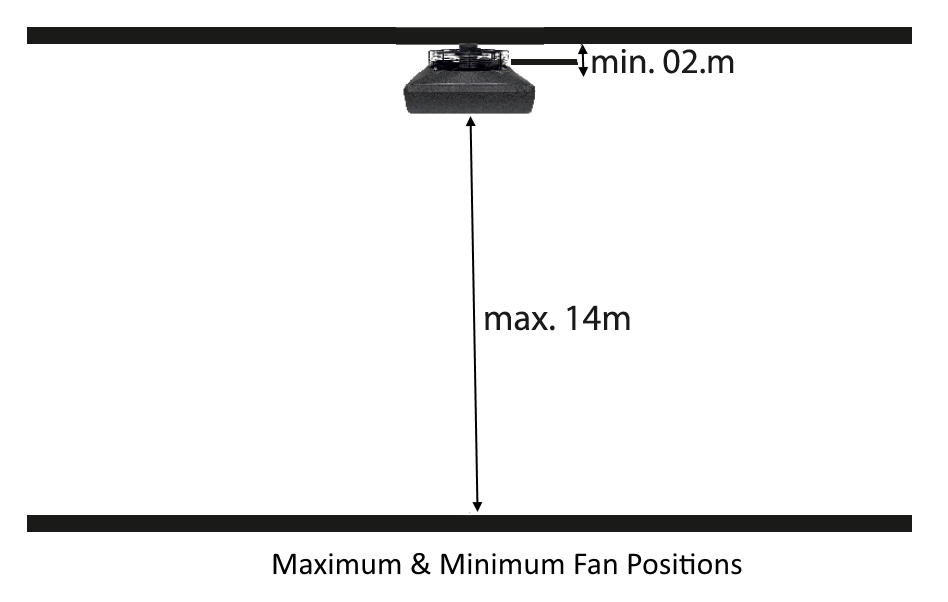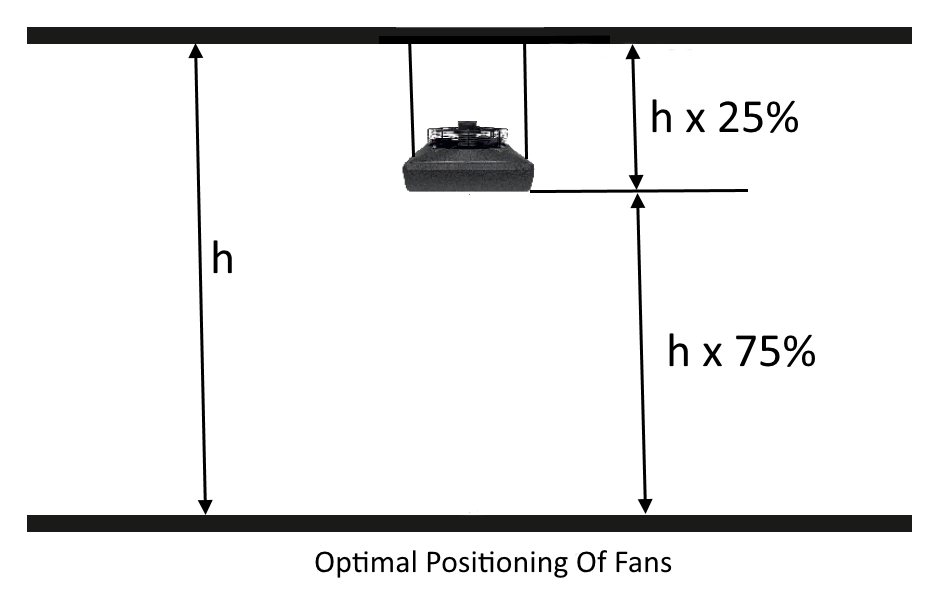14
November, 2017

One of the most common questions we get is “how many destratification fans do I need to properly utilise all the heat available in my building?”

The good thing is that it is reasonably simple to calculate. You just need to do the maths.

The first thing that needs to be understood is how a destratification fan works and what we are trying to achieve.

As a building heats up, the heat tends to rise and we end up with a situation where the high levels in the buildings are warmer than that at ground level.

If we want to maintain the heating levels in the working areas we have to keep on providing new heat to maintain the heat at ground level. Meanwhile the ceiling temperature continues to rise and much of this costly heat is lost through the roof.

The purpose of the destratification fan is to move this heat back to the ground level again thus reducing the need to introduce new heat. The effect of this process is to even out the heat throughout the space so that the temperatures throughout the space are all equal.

To maintain even heat throughout a space it is necessary to move the warm air above the destratification fans and mix it with the cooler air below.

The technical boffins at Reventon have carried out in depth studies of the most effective way of maximising the effectiveness of their destratification fans.

The have said that the optimal position for the fans is at a point ¾ of the distance from the floor to ceiling. For example, a building with a roof height of 10m should have the fans installed 2.5 metres below the ceiling.

Minimum distance from ceiling is 200mm and maximum distance from the floor is 14m. See illustrations below:Reventon recommend a fan capacity that will move the air above the destratification fan 6 times per hour based on the optimal fan position.

Now that we have established the basic principles, the calculations are carried out in 2 simple steps:

Step 1 – Calculate the volume of air above the fans at optimal positioning.

Example: A building with dimensions 150ft wide x 300ft long x 20ft height

Volume = 150 x 300 x 20 = 900,000 cubic feet

Convert to cubic metres = Divide by 35.32 = 25,481 cubic metres

25% of this is 6,370 cubic metres

Calculate total air to be moved by multiplying by 6 = 38,220 cubic metres

Step 2 – Calculate the Number of Reventon Destratification fans required.

Each fan moves 5,100 cubic metres per hour

Number of fans required = 38,220 divided by 5,100 = 7.49 – Rounded up to 8 fans.